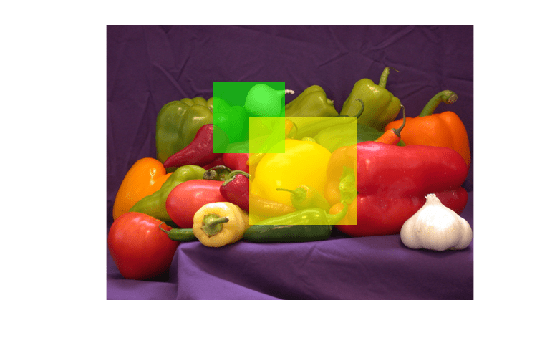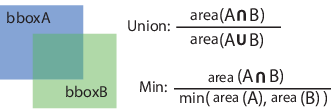Documentation

### This is machine translation

Mouseover text to see original. Click the button below to return to the English version of the page.

# bboxOverlapRatio

Compute bounding box overlap ratio

## Syntax

``overlapRatio = bboxOverlapRatio(bboxA,bboxB)``
``overlapRatio = bboxOverlapRatio(bboxA, bboxB, ratioType)``

## Description

example

````overlapRatio = bboxOverlapRatio(bboxA,bboxB)` returns the overlap ratio between each pair of bounding boxes `bboxA` and `bboxB`. The function returns the `overlapRatio` value between `0` and `1`, where `1` implies a perfect overlap.```
````overlapRatio = bboxOverlapRatio(bboxA, bboxB, ratioType)` additionally lets you specify the method to use for computing the ratio. You must set the `ratioType` to either `'Union'` or `'Min'`.```

## Examples

collapse all

Define two bounding boxes in the format [x y width height].

```bboxA = [150,80,100,100]; bboxB = bboxA + 50;```

Display the bounding boxes on an image.

```I = imread('peppers.png'); RGB = insertShape(I,'FilledRectangle',bboxA,'Color','green'); RGB = insertShape(RGB,'FilledRectangle',bboxB,'Color','yellow'); imshow(RGB)```Compute the overlap ratio between the two bounding boxes.

`overlapRatio = bboxOverlapRatio(bboxA,bboxB)`
```overlapRatio = 0.0833 ```

Randomly generate two sets of bounding boxes.

```bboxA = 10*rand(5,4); bboxB = 10*rand(10,4);```

Ensure that the width and height of the boxes are positive.

```bboxA(:,3:4) = bboxA(:,3:4) + 10; bboxB(:,3:4) = bboxB(:,3:4) + 10;```

Compute the overlap ratio between each pair.

`overlapRatio = bboxOverlapRatio(bboxA,bboxB)`
```overlapRatio = 5×10 0.2431 0.2329 0.3418 0.5117 0.7972 0.1567 0.1789 0.4339 0.0906 0.5766 0.3420 0.1655 0.7375 0.5188 0.2786 0.3050 0.2969 0.4350 0.2477 0.2530 0.4844 0.3290 0.3448 0.1500 0.1854 0.4976 0.5629 0.4430 0.5027 0.2685 0.3681 0.0825 0.3499 0.0840 0.0658 0.5921 0.6498 0.1930 0.7433 0.0676 0.3752 0.1114 0.3114 0.0696 0.0654 0.5408 0.6234 0.2046 0.7557 0.0717 ```

## Input Arguments

collapse all

Bounding box, specified as an M-by-4 matrix. Each row of `bboxA` contains a vector in the format [x y width height], where x and y correspond to the upper left corner of the bounding box. Bounding boxes inputs `bboxA` and `bboxB` must be real, finite, and nonsparse.

Data Types: `single` | `double` | `int8` | `int16` | `int32` | `uint8` | `uint16` | `uint32`

Bounding box, specified as an M-by-4 matrix. Each row of `bboxB` contains a vector in the format [x y width height], where x and y correspond to the upper left corner of the bounding box. Bounding boxes inputs `bboxA` and `bboxB` must be real, finite, and nonsparse.

Data Types: `single` | `double` | `int8` | `int16` | `int32` | `uint8` | `uint16` | `uint32`

Ratio type, specified as the character vector `'Union'` or `'Min'`.

• Set the ratio type to `'Union'` to compute the ratio as the area of intersection between `bboxA` and `bboxB`, divided by the area of the union of the two.

• Set the ratio type to `'Min'` to compute the ratio as the area of intersection between `bboxA` and `bboxB`, divided by the minimum area of the two bounding boxes.Data Types: `char`

## Output Arguments

collapse all

Overlap ratio between two bounding boxes, returned as an M-by-N matrix. Each (I, J) element in the output matrix corresponds to the overlap ratio between row I in `bboxA` and row J in `bboxB`. The function returns `overlapRatio` in the between 0 and 1, where `1` implies a perfect overlap. If either `bboxA` or `bboxB` is double, then the function returns `overlapRatio` as `double`. Otherwise, the function returns it as `single`.

The function computes the overlap ratio based on the ratio type. You can set `ratioType` to `'Union'` or `'Min'`:Data Types: `single` | `double`